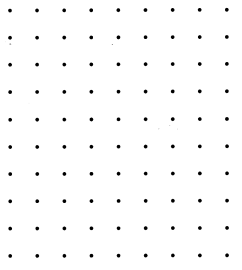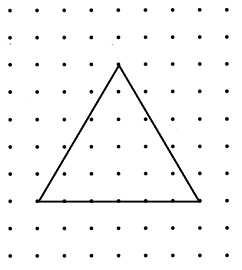# Math in Focus Grade 2 Chapter 19 Practice 1 Answer Key Plane Shapes

This handy Math in Focus Grade 2 Workbook Answer Key Chapter 19 Practice 1 Plane Shapes detailed solutions for the textbook questions.

## Math in Focus Grade 2 Chapter 19 Practice 1 Answer Key Plane Shapes

Look at the shapes.

Question 1.
Color the circles green, the triangles yellow, the rectangles purple, the trapezoids blue, the hexagons red, and the pentagons orange.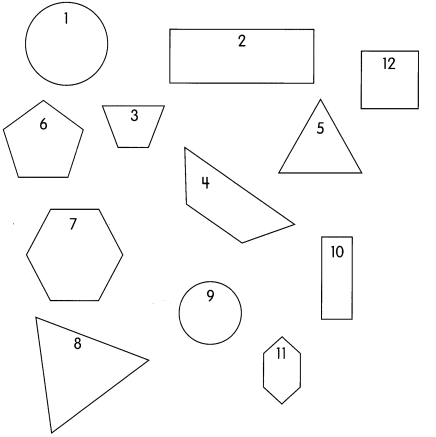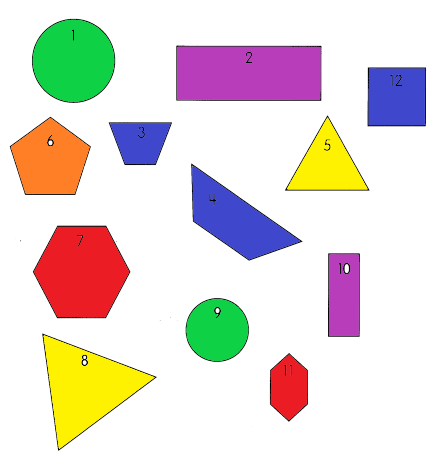Explanation:
I colored the circles green, the triangles yellow, the rectangles purple, the trapezoids blue, the hexagons red, and the pentagons orange.
There are 2 circles, 2 rectangles, 2 hexagons, 3 trapezoids, 2 triangles and 1 pentagon.

Question 2.
Which of these are quadrilaterals? Write the numbers: _____________
2, 4, 10 and 12 are quadrilaterals.

Draw lines on each shape to show the smaller shapes.

Example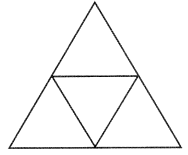Question 2.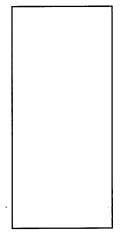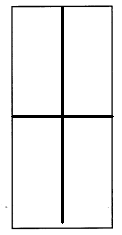Explanation:
I drew 2 lines to make smaller shapes in the given rectangle.

Question 3.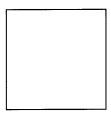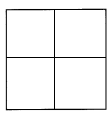Explanation:
I drew 2 lines to make smaller shapes in the given square.

Question 4.
Cut out the shapes. Then glue them on top of the shape given. Here are two simple rules to follow: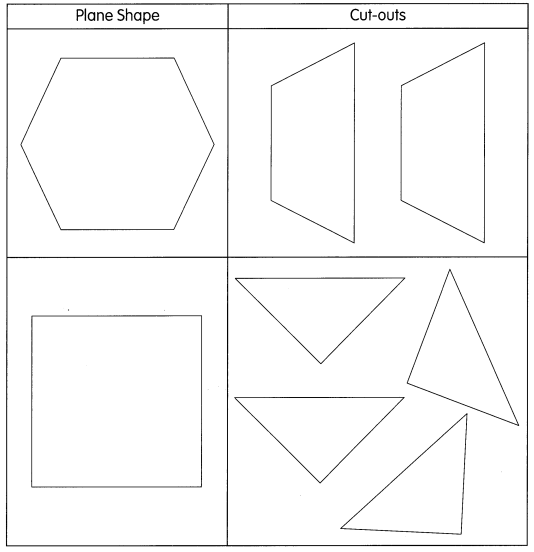a) All cut-outs must be used.

b) Cut-outs cannot overlap.

Question 5.
Draw lines on each figure to show how it is made with these shapes: triangle, square, rectangle, trapezoid, hexagon, and pentagon.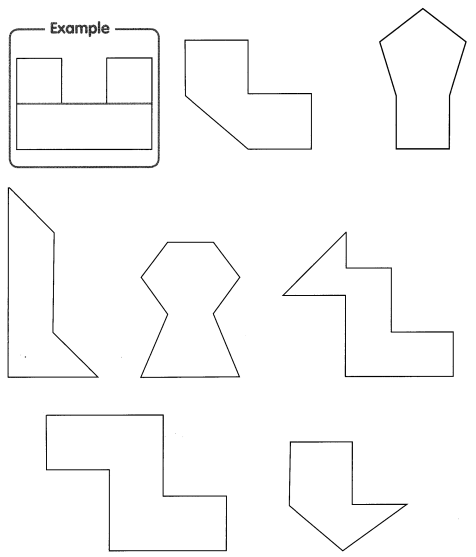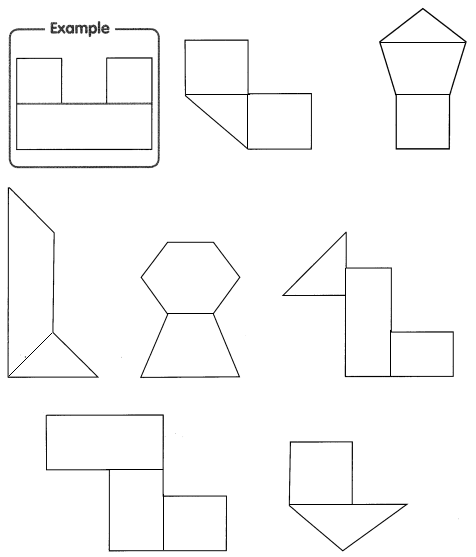Explanation:
I drew lines on each figure to show how it is made with these shapes: triangle, square, rectangle, trapezoid, hexagon, and pentagon.

Each figure is made with two shapes. Name the shapes.

Example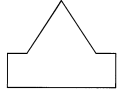The figure is made with a triangle and a rectangle.

Question 6.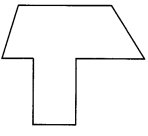The figure is made with a ___________ and a ___________.
The figure is made with a trapezium a and a rectangle.

Question 7.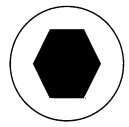The figure is made with a ___________ and a ___________.
The figure is made with a hexagon and a circle.

Question 8.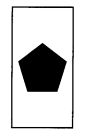The figure is made with a ___________ and a ___________.
The figure is made with a pentagon and a rectangle.

A part is missing from each figure. Color the shape that makes the figure complete.

Example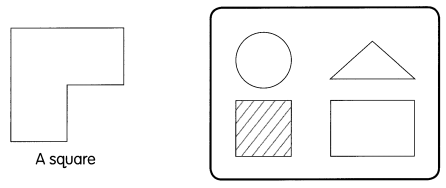Question 9.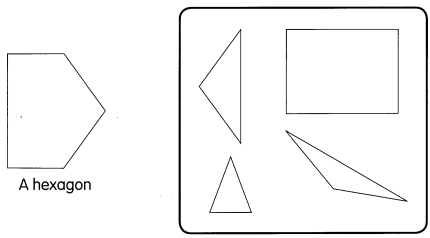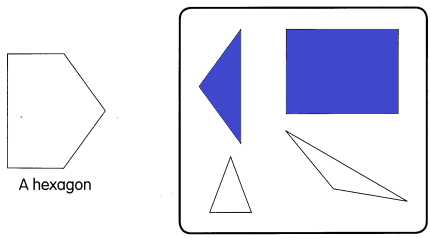Explanation:
A Hexagon is made up of a square and a rectangle.

A part is missing from each figure. Color the shape that makes the figure complete.

Question 10.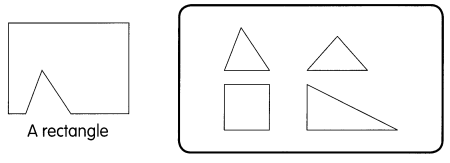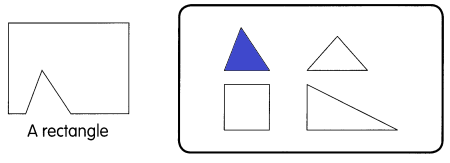Explanation:
The missing part in the above shape is a triangle
So, i colored the missing part.

Question 11.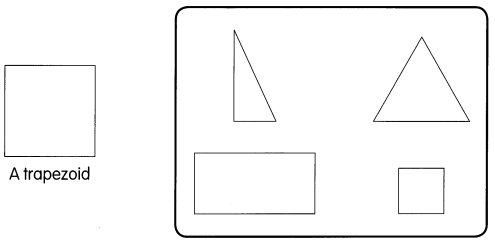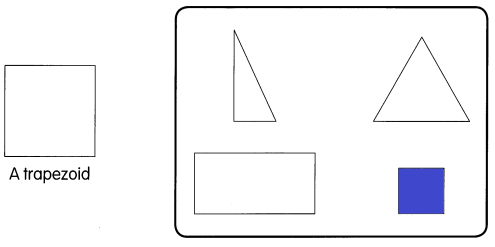Explanation:
A square is always a trapezoid
So, i colored square.

Question 12.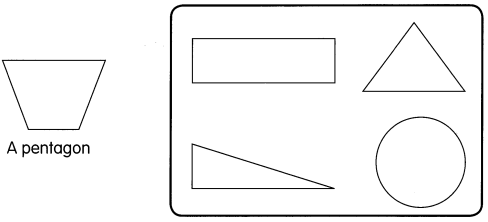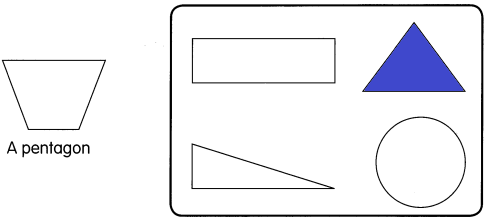Explanation:
To make a pentagon we need to join a triangle to a quadrilateral
So, i colored triangle.

Question 13.
Cut out the shapes. Then glue them on top of the figure.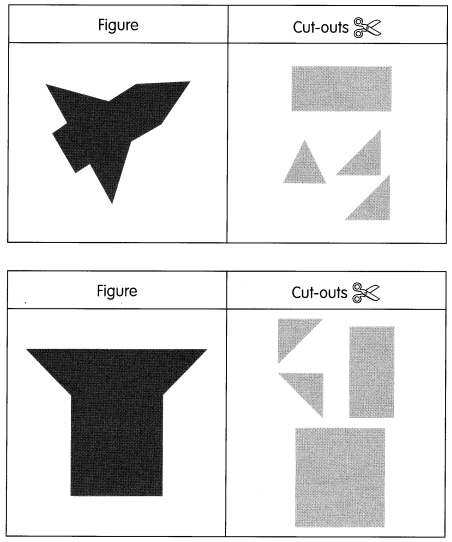Here are two simple rules to follow.
(a) All cut-outs must be used.

(b) Cut-outs cannot overlap.

Copy each figure.

Example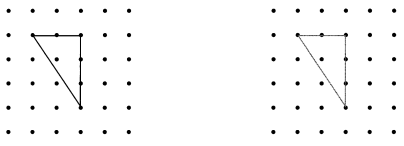Question 14.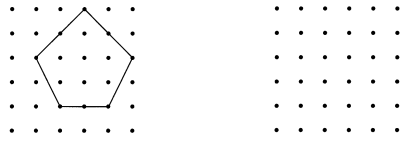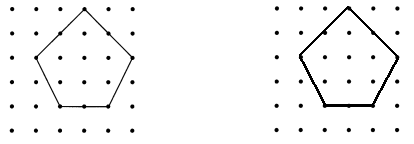Explanation:
I drew the same shape as shown in the first grid.
It is a pentagon.

Copy each figure.

Example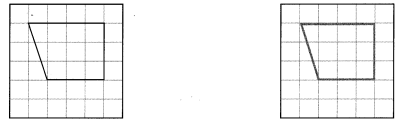Question 15.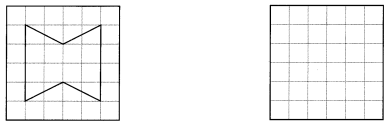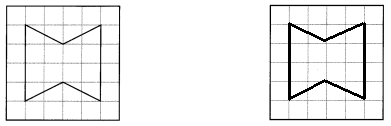Explanation:
I drew the same shape as shown in the first grid.
It is a Hexagon.

Draw a quadrilateral on the dot grid paper. Circle the angles. Write how many angles.

Question 16.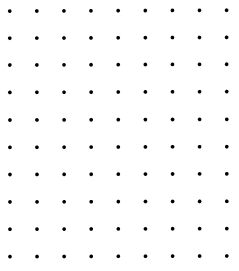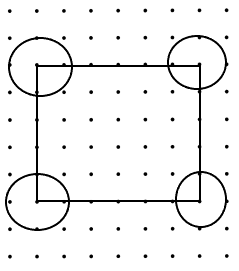Explanation:
I drew a rectangle and circled all the angles
A rectangle has 4 angles.

Draw a shape with three angles on the dot grid paper.

Question 17.